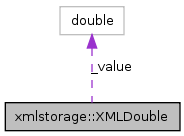# xmlstorage::XMLDouble Class Reference

type converter for numeric data More...

Collaboration diagram for xmlstorage::XMLDouble:[legend]

List of all members.

## Public Member Functions

this (double value)
this (in char[] value, double def=0.)
this (XMLNode node, char[] attr_name, double def=0.)
double opCast ()
char[] str ()

double _value

## Private Member Functions

void opAssign (XMLDouble)

## Detailed Description

type converter for numeric data

Definition at line 1407 of file xmlstorage.d.

## Constructor & Destructor Documentation

 xmlstorage::XMLDouble::this ( double value ) ` [inline]`

Definition at line 1410 of file xmlstorage.d.

```    {
_value = value;
}
```
 xmlstorage::XMLDouble::this ( in char[] value, double def = `0.` ) ` [inline]`

Definition at line 1415 of file xmlstorage.d.

```    {
if (value && *value)
_value = toDouble(value);
else
_value = def;
}
```
 xmlstorage::XMLDouble::this ( XMLNode node, char[] attr_name, double def = `0.` ) ` [inline]`

Definition at line 1423 of file xmlstorage.d.

```    {
char[] value = node.get(attr_name);

if (value.length)
_value = toDouble(value);
else
_value = def;
}
```

## Member Function Documentation

 void xmlstorage::XMLDouble::opAssign ( XMLDouble ) ` [private]`
 double xmlstorage::XMLDouble::opCast ( ) ` [inline]`

Definition at line 1433 of file xmlstorage.d.

```    {
return _value;
}
```
 char [] xmlstorage::XMLDouble::str ( ) ` [inline]`

Definition at line 1438 of file xmlstorage.d.

References xmlstorage::XS_FLOATFMT.

```    {
return format(XS_FLOATFMT, _value);
}
```

## Member Data Documentation

 double xmlstorage::XMLDouble::_value` [protected]`

Definition at line 1444 of file xmlstorage.d.

The documentation for this class was generated from the following file: